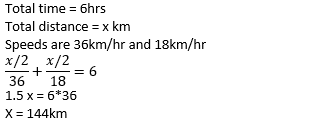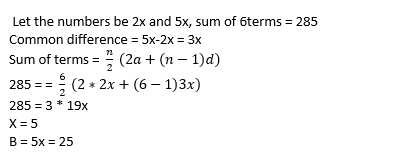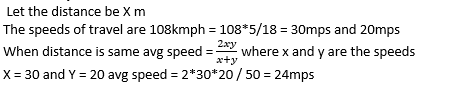# Hexaware Numerical Ability Previous Year Questions

04 January 2023

Hexaware Numerical Ability Previous Year Questions

Hexaware Numerical Ability Previous Year Questions

Q1) In how many ways can a cricket team of 11 players be selected out of 16 players, if two particular player are always to be included?

A. 2008

B. 2004

C. 2006

D. None of these

Explanation: No.of ways of selecting two particular players = 1 ways

And remaining 9 player from 14 (remaining after 2 players) = 14c9 = 2002

Therefore total no.of ways = 2002+1 = 2003 ways

Q2) A tells B “If you give me Rs 400, then | shall have 25% more than what you have”. B tells A, “If you give me Rs 200, then | shall have three-and-a-half times as much as you have.” How much Rupees B have?

A. Rs 1600

B. Rs 800

C. Rs 900

D. Rs 1200

Explanation: Let amount of A = X and B = Y

If you give me Rs 400, then | shall have 25% more than what you have

If B give him 400 then A = x+400 and B =Y -400

X+400 = (Y-400) + 25% of (Y-400)

4X+1600 = 5Y – 2000

5Y-4X = 3600

B tells A, “If you give me Rs 200, then | shall have three-and-a-half times as much as you have.”

Then  Y +200 = 7/2 ( X-200)

2Y +400 = 7X -1400

7X -2Y = 1800

Solving both Y = 1200/-

Q3) Aman invests Rs. 10,000 in some shares in the ratio 2:3:5 which pay dividends of 10%, 25% and 20% (on his investment) For that year respectively. Find his dividend income.

A. 2050

B. 1950

C. 2000

D. 1900

Explanation: According to the given question the man invests rupees 10000 in some shares in the ratio 2:3:5.

That means the share amount will be 2000, 3000 and 5000 respectively.

It is also said that the dividend is 10%, 25% and 20% respectively on the investment.

Therefore, the dividend amount will be 200, 750 and 1000. [10% of 2000, 25% of 3000 and 20% of 5000]

There for his total dividend income will be the sum of the three dividends that is 200 + 750 + 1000 which is equal to Rs 1950. [Ans]

Q4) The length, breadth and height of a room are in the ratio, 3 : 2: 1. If the breadth and height are halved and the length is doubled, then the total area of the four walls of the room will

A. decrease by 15%

B. decrease by 30%

C. remain the same

D. decrease by 13.64%

Explanation: Let l=3x, b=2x, h=x

Area of four walls =2(l+b)h=2(3x+2x)x=10x2

L1=6x , b1=x/2 , h=x/2

New area = 7x2

% decrease = 3 x2 / 10 x2  *100 = 30%

Q5) If 4 examiners can examine a certain number of answer books in 8 days by working 5 hours a day, for how many hours a day would 2 examiners have to work in order to examine twice the number Of error

A. 8

B. 6

C. 9

D. 5

Explanation:

(m1*d1*h1)/w1 = (m2*d2*h2)/w2

assume w = 100;

(4*8*5)/100=(2*20*h)/200

h = 8 hrs

Q6) Mitchell travels for a total of 6 hours. If he travelled the first half of the distance at 36  kph and the second half at 5 mps, find the total distance traveled by him in km.

A.143

B.196

C.164

D.144

Explanation:Q7) A mixture of  three materials A, B and C (in powdered form) with the volumes in the ratio 4:2:3 is required to make good quality powder. The weights of 1 m3 of A, B  and C are 90 kg, 100 kg and 120 kg respectively. What is the ratio of the weights of A and B to B and C in the mixture?

A.2 : 3

B.1 : 2

C.1 : 1

D.2 : 1

Explanation: The weights of 1 m3 of A, B and C are 90 kg, 100 kg and 120 kg respectively

A = 90* 4 = 360m3

B = 100*2 = 200m3

C = 120*3 =360m3

ratio of the weights of A and B to B and C in the mixture

(360+200) : (200+360)

1:1

Q8) A man is running at  a speed of 2mps. There is a bus stop of length 10m at a distance of 80m from the man. What is the time required by the man to pass by the bus stop?

A.45 seconds

B.19 seconds

C.34 seconds

D.24 seconds

Explanation: Speed = 2mps

Distance = 10+80 = 90m

Time = 90/2 = 45sec

Q9) If sinA and cosA are the roots of the  equation ax2 + bx + c = 0, then which of the following relationship is correct?

A.(a - c)2 = b2 + c2

B.(a + c)2 = b2 - c2

C.(a - c)2 = b2 - c2

D.(a + c)2 = b2 + c2

Explanation: Sum of roots

SinA +cosA = -b/a ---- (1)

Product

sinA*cosA = c/a ----(2)

squaring on both sides for 1

(SinA +cosA)2 = b2 / a2

Sin2A + cos2A + 2* sinA*cosA = b2 / a2

1+2c/a = b2 / a2

a2+2ac = b2

adding c2 on both sides then option D follows.

Q10) The monthly income of A, B and C  in the ratio 2:3:1 and the monthly income of B, C and D are in the  ratio 9:3:15. The saving of  C and D are in the ratio 2:3 and C saves \$600. What  is the annual saving of D, if monthly income of A is \$6000?

A.\$9800

B.\$11500

C.\$10800

D.\$8500

Explanation: The monthly income of A, B and C in the ratio 2:3: 1, and income of B, C, D is 3:1:5

Therefore, ratio of A, B, C and D will be 2:3:1:5

A = 6000 then D = 15000

Saving of C = 600/-

Ratio of saves of C and D = 2:3

Saving of D = 900 per month, annual saving = 900*12 = \$10800

Q11) The  ratio of two numbers A and B is 2 : 5 and the sum of 6 terms of arithmetic progression starting with A and common difference(B - A) is 285. Find the value of B.

A.25

B.30

C.20

D.15

Explanation:Q12) Cameron covered a certain distance at 108 kph and returned back at 20 mps. Find the average speed in mps for the entire journey.

A.32

B.25

C.24

D.18

Explanation:Q13) The ratio of salaries of Peter and Paul was 2:3. Their salaries were increased by Rs. 200 and Rs. 600 respectively, and the new ratio became 1:2. What were their salaries initially?

A.Rs. 600, Rs. 900

B.Rs. 200, Rs. 300

C.Rs. 800, Rs. 1200

D.Rs. 400, Rs. 600

Explanation: The monthly incomes of Peter and Paul are in the ratio of 2: 3

Let the monthly income of peter = 2x

Let the monthly income of paul = 3x

New salaries will be

Peter = 2x+200 , Paul = 3x+600

Therefore

2x +200 / 3x+600 = 1/2

4x+400 = 3x+600

X = 200

Salaries will be 400 and 600 respectively.

Q14) Emma invested \$5200 under simple interest at 6% per annum. Find the simple interest earned by her after 3 years?

A.\$956

B.\$936

C.\$846

D.\$898

Explanation: P= 5200, r = 6%, T = 3years.

S.I = PTR /100 = 5200*6*3 / 100 = \$936

Q15) Pipe M can fill a tank in 3 hours, and pipe N can fill the tank in 2 hours. If they are opened simultaneously, in how much times (in hours) can they fill it?

A.1.2

B.1.5

C.2.4

D.3.6

Explanation: Let the capacity be 6 lits

Pipe M can fill a tank in 3 hours, hence the efficiency = 2 lit/hr

Pipe N can fill a tank in 2 hours, hence the efficiency = 3 lit/hr

If the pipes are open simultaneously then 5lits will be filled in 1hrs.

Therefore, 6 lits can be filled in 1.2 hr

##